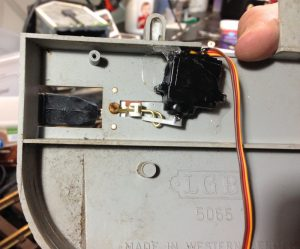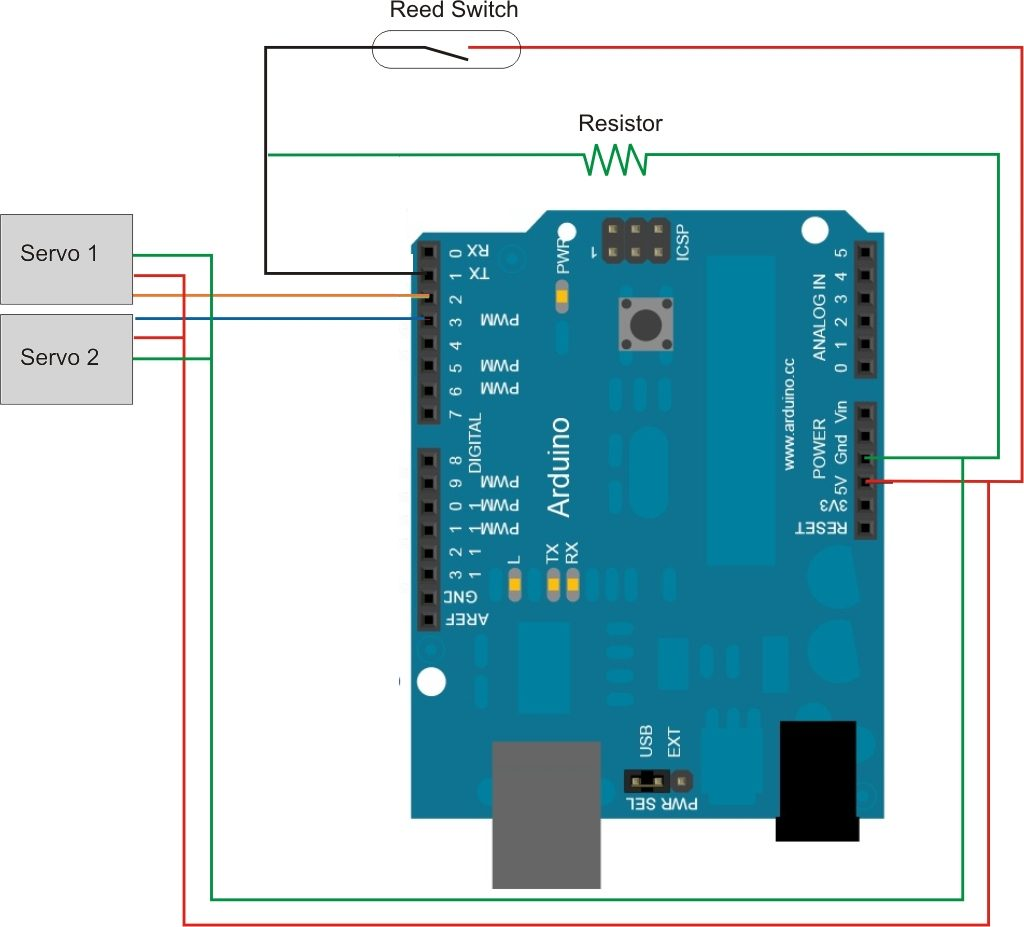# Servo Activated LGB Rail Crossing

I’ve been fiddling with this for quite some time. My initial experiments didn’t work out too well as the IR sensors didn’t like the waterproofing and the “stainless” steel wire I was using rusted out.

But here’s some progress

The servos are mounted in the bottom of a set of LGB crossing gates.The servos are controlled by an Arduino board.

The program is set up to close the gates when a trigger is sensed (currently a reed switch). Then it starts counting to three seconds and monitors the switch. If another trigger occurs within the three seconds, the timer starts over. Once no trigger has been sensed for three seconds, the gates raise.

Here’s a circuit drawingHere’s the Arduino sketch

```// include the servo header file
#include <Servo.h>

// create servo object to control a servo
Servo myservo1;
Servo myservo2;

// constants for pins
const int buttonPin = 1;
const int servo1Pin = 2;
const int servo2Pin = 3;
const int ledPin = 13;

// variables
boolean gateClosed = false;
int buttonState = 0;
int pos = 80;
int oppos = 0;
long detectTime;
long t;

//setup routine
void setup(){
// initialize the LED pin as an output:
pinMode(ledPin, OUTPUT);
// initialize the pushbutton pin as an input:
pinMode(buttonPin, INPUT);
// set the LED to off
digitalWrite(ledPin, LOW);
// initialize the servo pin as a servo
myservo1.attach(servo1Pin);
myservo2.attach(servo2Pin);
}

// main program loop
void loop(){
// if the button state is HIGH then the switch is closed
if (buttonState == HIGH){
// set time of switch closed detection
detectTime=millis();
//call the closeGate subroutine
closeGate();
}
// set t to the value of current time minus
// switch detection time
t= millis()-detectTime;
//check if it has been more than 4 seconds since the
// last switch closed detection
if (gateClosed==true && t>=3500 ){
// call the openGate subroutine
openGate();
}
}

// subroutine to close the gates
void closeGate(){
// only run if the gate state is open
if (gateClosed==false){
//set the LED "on" for visual feedback'
digitalWrite(ledPin, HIGH);
//run the servo to the closed position
for (pos = 80; pos >= 0; pos -= 1) {
myservo1.write(pos);
oppos = (pos*1.375);
myservo2.write(oppos);
delay(30);
}
}
//set the gate state to closed
gateClosed=true;
}

// subroutine to open the gates
void openGate(){
// only run if the gate state is open
if (gateClosed==true){
//set the LED "on" for visual feedback'
digitalWrite(ledPin, LOW);
//run the servo to the open position
for (pos = 0; pos <= 80; pos += 1) {
myservo1.write(pos);
oppos = (pos*1.375);
myservo2.write(oppos);
delay(30);
}
}
//set the gate state to open
gateClosed=false;
}```